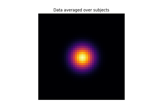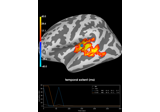# mne.stats.spatio_temporal_cluster_1samp_test¶

mne.stats.spatio_temporal_cluster_1samp_test(X, threshold=None, n_permutations=1024, tail=0, stat_fun=None, adjacency=None, n_jobs=1, seed=None, max_step=1, spatial_exclude=None, step_down_p=0, t_power=1, out_type='indices', check_disjoint=False, buffer_size=1000, verbose=None)[source]

Non-parametric cluster-level paired t-test for spatio-temporal data.

This function provides a convenient wrapper for `mne.stats.permutation_cluster_1samp_test()`, for use with data organized in the form (observations × time × space). See 1 for details.

Parameters
X`array`, shape (n_observations, n_times, n_vertices)

The data to be clustered. The first dimension should correspond to the difference between paired samples (observations) in two conditions.

threshold

If numeric, vertices with data values more extreme than `threshold` will be used to form clusters. If threshold is `None`, a t-threshold will be chosen automatically that corresponds to a p-value of 0.05 for the given number of observations (only valid when using a t-statistic). If `threshold` is a `dict` (with keys `'start'` and `'step'`) then threshold-free cluster enhancement (TFCE) will be used (see the TFCE example and 2).

n_permutations`int` | ‘all’

The number of permutations to compute. Can be ‘all’ to perform an exact test.

tail`int`

If tail is 1, the statistic is thresholded above threshold. If tail is -1, the statistic is thresholded below threshold. If tail is 0, the statistic is thresholded on both sides of the distribution.

stat_fun

Function called to calculate the test statistic. Must accept 1D-array as input and return a 1D array. If `None` (the default), uses `mne.stats.ttest_1samp_no_p()`.

Defines adjacency between locations in the data, where “locations” can be spatial vertices, frequency bins, etc. If `False`, assumes no adjacency (each location is treated as independent and unconnected). If `None`, a regular lattice adjacency is assumed, connecting each spatial location to its neighbor(s) along the last dimension of `X`. If `adjacency` is a matrix, it is assumed to be symmetric (only the upper triangular half is used) and must be square with dimension equal to `X.shape[-1]` (n_vertices) or `X.shape[-1] * X.shape[-2]` (n_times * n_vertices). If spatial adjacency is uniform in time, it is recommended to use a square matrix with dimension `X.shape[-1]` (n_vertices) to save memory and computation, and to use `max_step` to define the extent of temporal adjacency to consider when clustering.

n_jobs`int`

The number of jobs to run in parallel (default 1). Requires the joblib package.

seed`None` | `int` | instance of `RandomState`

If `seed` is an `int`, it will be used as a seed for `RandomState`. If `None`, the seed will be obtained from the operating system (see `RandomState` for details). Default is `None`.

max_step`int`

Maximum distance along the second dimension (typically this is the “time” axis) between samples that are considered “connected”. Only used when `connectivity` has shape (n_vertices, n_vertices).

spatial_exclude

List of spatial indices to exclude from clustering.

step_down_p`float`

To perform a step-down-in-jumps test, pass a p-value for clusters to exclude from each successive iteration. Default is zero, perform no step-down test (since no clusters will be smaller than this value). Setting this to a reasonable value, e.g. 0.05, can increase sensitivity but costs computation time.

t_power`float`

Power to raise the statistical values (usually t-values) by before summing (sign will be retained). Note that `t_power=0` will give a count of locations in each cluster, `t_power=1` will weight each location by its statistical score.

Output format of clusters within a list. If `'mask'`, returns a list of boolean arrays, each with the same shape as the input data (or slices if the shape is 1D and adjacency is None), with `True` values indicating locations that are part of a cluster. If `'indices'`, returns a list of tuple of ndarray, where each ndarray contains the indices of locations that together form the given cluster along the given dimension. Note that for large datasets, `'indices'` may use far less memory than `'mask'`. Default is `'indices'`.

check_disjointbool

Whether to check if the connectivity matrix can be separated into disjoint sets before clustering. This may lead to faster clustering, especially if the second dimension of `X` (usually the “time” dimension) is large.

buffer_size

Block size to use when computing test statistics. This can significantly reduce memory usage when n_jobs > 1 and memory sharing between processes is enabled (see `mne.set_cache_dir()`), because `X` will be shared between processes and each process only needs to allocate space for a small block of locations at a time.

verbose

If not None, override default verbose level (see `mne.verbose()` and Logging documentation for more). If used, it should be passed as a keyword-argument only.

Returns
t_obs`array`, shape (n_times * n_vertices,)

T-statistic observed for all variables.

clusters`list`

List type defined by out_type above.

cluster_pv`array`

P-value for each cluster.

H0`array`, shape (n_permutations,)

Max cluster level stats observed under permutation.

References

1

Eric Maris and Robert Oostenveld. Nonparametric statistical testing of EEG- and MEG-data. Journal of Neuroscience Methods, 164(1):177–190, 2007. doi:10.1016/j.jneumeth.2007.03.024.

2

Stephen M. Smith and Thomas E. Nichols. Threshold-free cluster enhancement: addressing problems of smoothing, threshold dependence and localisation in cluster inference. NeuroImage, 44(1):83–98, 2009. doi:10.1016/j.neuroimage.2008.03.061.

## Examples using `mne.stats.spatio_temporal_cluster_1samp_test`¶